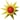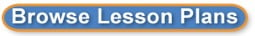Search results for equations:
 Browse All Lesson Plans Lesson Plan Name Grades Equations in Motion 8 to 11(0 stars, 1 ratings)Groups of two or three students will walk around the Block taping items in motion and then using the Vernier Video Physics App write equations for the motion they see. Tis will be a culminating activity for our unit on writing equations. Graphing quadratic equations of the form f(x) = ax^2 + c 9 to 12(0 stars, 1 ratings)In this lesson, we explore the effect of the constant C in the quadratic function f(x) = ax^2. Students will be able to observe that C shifts the quadratic function up/down. Quadratic Equations in Action 9 to 12(0 stars, 1 ratings)Students will video and analyze real-life situations that produce a parabolic curve. Ratio/proportional Relationships: using graphs, tables, and equations 6 to 7(0 stars, 1 ratings)Students will match proportional relationships using graphs, tables, and one-step equations to show hoe they are related. Real World Equations! 9 to 12(0 stars, 1 ratings)Students will use digital cameras to take photos of items or situations that can be modeled using algebraic equations. Solving Systems of Linear Equations Using Substitution 7 to 12(0 stars, 1 ratings)This Algebra I lesson uses SMART Board technology to provide students with a physical sense of the process of substitution and how it can be used in solving a system of linear equations. Capturing Conic Sections with Digital Cameras 9 to 12(0 stars, 2 ratings)This project will help students to make connections between math and the real world by having them identify conic sections in art and architecture in their communities. Students will photograph these examples of circles, ellipses, parabolas, and hyperbolas in the real world with digital cameras and then write the equations for the graphs. Chemical Reaction Demonstration 9 to 12(0 stars, 2 ratings)Identify and classify chemical reactions based on the five following types of reactions: synthesis, decomposition, single replacement, double replacement, and combustion. I Spy 9 to 12(0 stars, 1 ratings)Students use digital cameras to find objects in and around school that have the shape of various functions, conics, and graphs that they have learned. Students will also write an equation that could represent the shape. Parallel and Perpendicular lines in our world 9 to 11(0 stars, 1 ratings)The students will collect real world examples of parallel and perpendicular lines and take pictures of them. Then the students will add a coordinate grid to the pictures and calculate the equations of the lines. Finally, the students will put together a presentation of their pictures, equations, and explanation of how the lines are related. Podcasting Parabolas 7 to 12(0 stars, 1 ratings)After an introductory lesson on parabolas, students will research parabolas, the general equation of a parabola from three points and photograph pictures of parabolas found in everyday life. Students will then organize the data to create and publish a podcast to be share with their peers in the classroom, as well as, around the world. (This is a 3-day lesson for the block schedule) Seeing Math Everywhere We Look 9 to 9(0 stars, 2 ratings)Through an exploration and photographic capture of parabolas throughout our environment. students will move from the When am I ever going to use this? to I see it being used stage in math class  specifically for quadratic equations. They will then research the related jobs necessary to "bring the parabola to life." The Algebra of Angry Birds© 6 to 8(0 stars, 2 ratings)Angry Birds is a popular application (app) that features birds that are launched from a slingshot at green pigs. Students will explore algebraic and physics content that is embedded in the game. "50 Ways to Use Your FlipCam" 9 to 12(0 stars, 1 ratings)This lesson/power point was developed in order to teach the audience (teachers/instructors) simple and quick ways to enhance their teaching and to help invest their student in their education by using a FlipCam. 3D printing for Math and for projects 6 to 12(0 stars, 1 ratings)The ability to figure out the equations necessary to print basic shapes and the ability to print useful items needed for robotics, drama productions, math/history/geography/all subjects demonstrations would be amazing!! 3D Printing in Algebra Class? P-K to 8(0 stars, 2 ratings)As a math teacher, one of the most common questions that students have is "Why do we have to learn this?" When students realize that they will need to apply algebraic reasoning to design and print something in 3D, their motivation and interest in mathematics will increase exponentially. A Matter of Fact 5 to 6(0 stars, 2 ratings)A math and science unit on matter. Discovering Strategies to Divide 5 to 5(0 stars, 1 ratings)Students will solve real life story problems by modeling, writing equations, and justifying their use of operations and strategies. Strategies and solutions are then shared with the whole group to encourage flexible math thinking. Growing STEM Minds Through the Growing Gardens 9 to 12(0 stars, 2 ratings) The nexus of our STEM activities revolves around our urban gardening center surrounding the school. The STEM activities will reach across all the subject areas including English, History, Science, Engineering, Technology and Math classes at our school. How Much does it Cost Project 7 to 9(0 stars, 2 ratings)In this project, students investigate pricing schemes for various entry fees and determine which is the better option depending on their situation. Interpret the equation 8 to 8(0 stars, 1 ratings)To interpret the equation of line, students will rotate through three stations. Each station will require the students to interpret the equation but using different techniques. Jumping in the air - What was your height? 7 to 8(0 stars, 1 ratings)Using video equipment and quadratic formulas students will determine the height of their jumps. Linear Relationships in the Real World 9 to 12(0 stars, 1 ratings)The student will use problem solving, mathematical communication, mathematical reasoning, connections and representations to solve multi-step equations in one variable with the variable on one of two sides of the equations while identifying at least 3 careers which utilize this skill. Manipulating Graphs 7 to 8(0 stars, 1 ratings)This lesson will help students make a connection to the slope-intercept form of a graph, y=mx+b and how it relates to the real world. Multiplication and Division Strategy Podcast 3 to 5(0 stars, 1 ratings)The students will draft, edit, and publish a podcast explaining their favorite strategy for solving multiplication and division word problems. Multiplying 2-digit Numbers 4 to 4(0 stars, 1 ratings)Students will use several strategies to multiply two-digit numbers by two-digit numbers. They will use area models, partial products, and the standard algorithm. Paper Airplanes 9 to 12(0 stars, 2 ratings)Applications of Scatter Plots, Lines of Best Fit, Dependant & Independent Variables through the making and flying of paper airplanes. I have the class research several designs of airplanes, we discuss aerodynamics, construct a their desired model, fly the model, gather information and plot the results. Parabolas in Flight 9 to 12(0 stars, 2 ratings)Students will film a trajectory then calculate the quadratic model for their trajectory. They will create a video to display online at teachertube. Quadratics in Nature and Architecture 9 to 12(0 stars, 3 ratings)Students will discover real-life applications of quadratic functions using video cameras. The students will learn how to write equations for the parabolas that they find in real-life. Sea Scallop Data Mining Research Project 10 to 12(0 stars, 1 ratings)Students develop a research question and then gather the data to answer that question using the National Oceanic and Atmospheric Administration's Sea Scallop Survey database. Students present the results in a formal classroom presentation and a scientific poster session which is open to the public. Show What You Know-Solving Subtraction Problems (K/1st Grade) P-K to 5(0 stars, 1 ratings)The purpose of this lesson is to help students understand the concept behind the abstract symbols used in subtraction. Specifically, Kindergarteners are still learning number symbols and alphabets as well as the plus sign so distinguishing symbols and what they represent when presented with them are extremely important. All students need concept development to retain such skills. This lesson will teach students a new strategy for solving subtraction as well as provide a pictorial representation of subtraction. Language development of vocabulary like minus, take away, less and fewer is also important for all students in math progression as these terms will be used in word problems and comparing amounts throughout school and in the real world. First graders will have a combination of addition and subtraction with subtraction word problems. Students will discuss these concepts, learn and practice a new strategy and then use the strategy that works best for them in their independent and partner tasks. Upon completion of tasks some students will interview each other to discuss which strategy they used and why and how they used it. Others will create an avatar cartoon video or a song to share their strategy for solving subtraction problems. Solving 2-step Inequalities 7 to 8(0 stars, 1 ratings)Students/teachers can do entire lesson on line w/videos and examples to enhance learning. Stations: Equation of a line and Slope 8 to 8(0 stars, 1 ratings)This is a stations lesson to check the learner's understanding of graphing lines and slope. The learner will receive immediate feedback at each station to determine if they fully understand the concept. The Tales of Nerdy Norm 8 to 12(0 stars, 1 ratings)In this lesson, students will create a stop motion video that showcases the transformations of quadratic functions by creating a clay model of "Nerdy Norm the Normal Parabola." Students will add audio to their movie to tell the tale of how a shift in Nerdy Norm's "mood" changes his graph! Virtual Math Portfolio 7 to 9(0 stars, 1 ratings)Students will create a customized web page to post a series of unit-based math projects. They will keep a copy of the web page as a virtual portfolio of their exciting math year. Writing Equivalent Expressions 9 to 12(0 stars, 1 ratings)This involves writing equivalent expressions using area model and exploring difference of squares through an interactive activity.